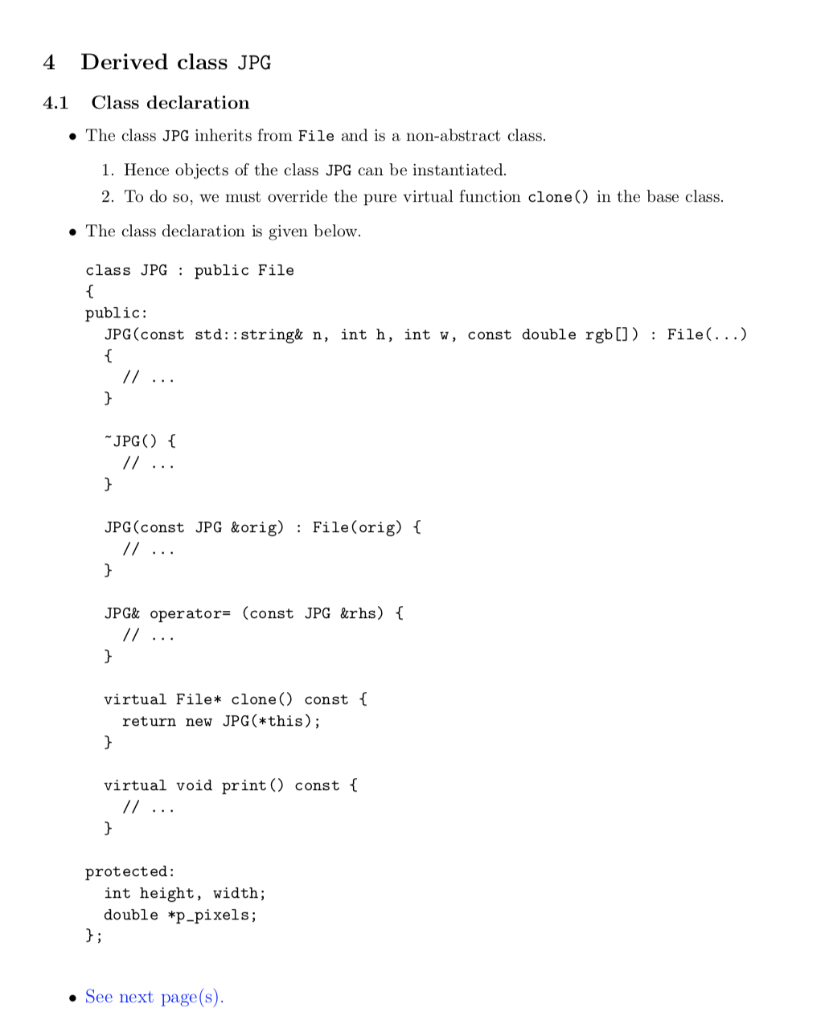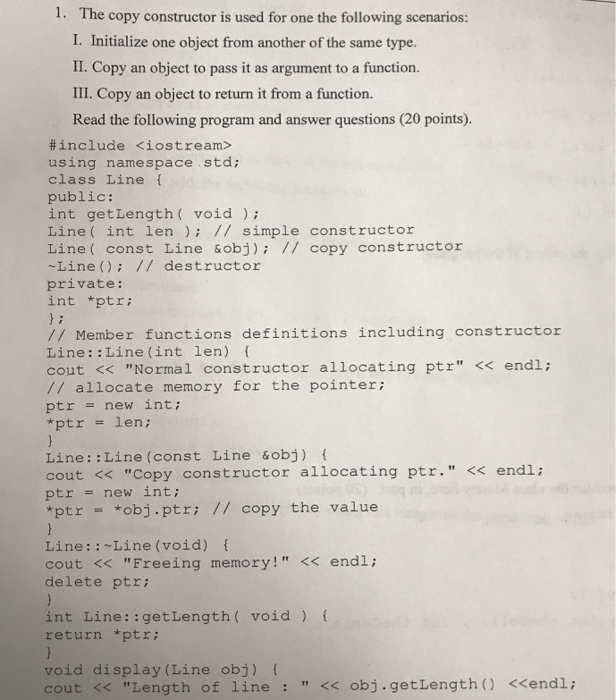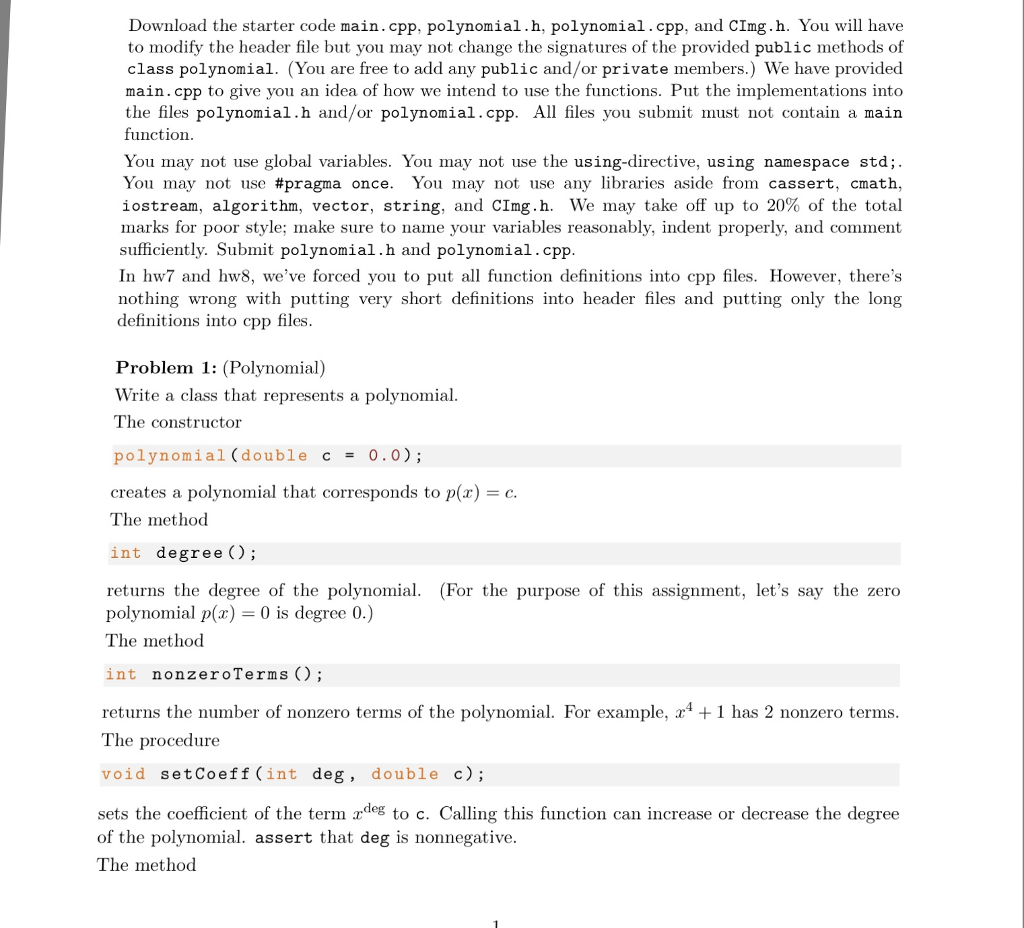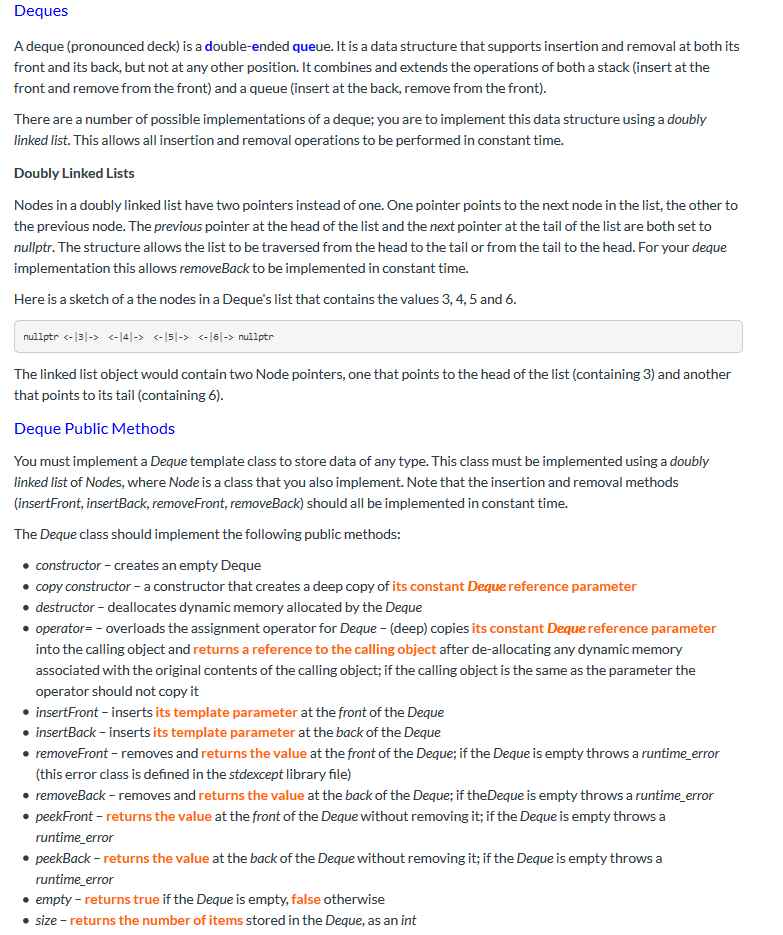Question

Multiple Choice

 Multiple Choice Section 4.1 Pointers and Dynamic Memory
1. Consider the following statements:
```    int *p;
int i;
int k;
i = 42;
k = i;
p = &i;
```
After these statements, which of the following statements will change the value of i to 75?
• A. k = 75;
• B. *k = 75;
• C. p = 75;
• D. *p = 75;
• E. Two or more of the answers will change i to 75.
2. Consider the following statements:
```   int i = 42;
int j = 80;
int *p1;
int *p2;
p1 = &i;
p2 = &j;
*p1 = *p2;
cout << i << j << endl;
```
What numbers are printed by the output statement?
• A. 42 and then another 42
• B. 42 and then 80
• C. 80 and then 42
• D. 80 and then another 80
3. What is printed by these statements?
```    int i = 1;
int k = 2;
int *p1;
int *p2;
p1 = &i;
p2 = &k;
p1 = p2;
*p1 = 3;
*p2 = 4;
cout << i;
```
• A. 1
• B. 2
• C. 3
• D. 4
4. What is printed by these statements?
```     int i = 1;
int k = 2;
int* p1;
int* p2;
p1 = &i;
p2 = &k;
p1 = p2;
*p1 = 3;
*p2 = 4;
cout << k;
```
• A. 1
• B. 2
• C. 3
• D. 4
5. What is printed by these statements?
```     int i = 1;
int k = 2;
int* p1;
int* p2;
p1 = &i;
p2 = &k;
*p1 = *p2;
*p1 = 3;
*p2 = 4;
cout << i;
```
• A. 1
• B. 2
• C. 3
• D. 4
6. When allocating an array of objects, what constructor is used to initialize all of the objects in the array?
• A. The automatic copy constructor.
• B. The constructor specified at the declaration.
• C. The default constructor.
• D. None of the above.
7. In which location do dynamic variables reside?
• A. The code segment.
• B. The data segment.
• C. The heap.
• D. The run-time stack.
 Multiple Choice Section 4.2 Pointers and Arrays as Parameters
8. When should a pointer parameter p be a reference parameter?
• A. When the function changes p, and you want the change to affect the actual pointer argument.
• B. When the function changes p, and you do NOT want the change to affect the actual pointer argument.
• C. When the function changes *p, and you want the change to affect the the object that is pointed at.
• D. When the function changes *p, and you do NOT want the change to affect the the object that is pointed at.
• E. When the pointer points to a large object.
9. Suppose you have the following function prototype and variable declaration:
```    void goop(int z[ ]);
int x;
```
Which is the correct way to call the goop function with x as the argument:
• A. goop(x);
• B. goop(x[ ]);
• C. goop(x);
• D. goop(&x);
• E. goop(&x[ ]);
10. Suppose that the goop function from the previous question changes the value of z. Does this change effect the value of the actual argument?
• A. Yes
• B. No
11. Here is a function declaration:
```    void goo(int* x)
{
*x = 1;
}
```
Suppose that a is an int* variable pointing to some integer, and *a is equal to zero. What is printed if you print *a after the function call goo(a)?
• A. 0
• B. 1
• C. address of a
• D. address of x
• E. None of the above
 Multiple Choice Section 4.3 The Bag ADT with a Dynamic Array
12. Here is a small function that uses the dynamic bag class from Chapter 4:
```    void quiz( )
{
bag::size_type i;   // Line 1
bag b;              // Line 2

b.insert(42);       // Line 3
i = b.size( );      // Line 4
cout << i;    // Line 5
}
```
When is the bag's dynamic array allocated?
• A. During the execution of Line 2.
• B. During the execution of Line 3.
• C. Just after Line 4 and before line 5.
• D. After Line 5.
13. Here is a small function that uses the dynamic bag class from Chapter 4:
```    void quiz( )
{
bag::size_type i;  // Line 1
bag b;             // Line 2

b.insert(42);      // Line 3
i = b.size( );     // Line 4
cout << i;   // Line 5
}
```
When is the bag's dynamic array returned to the heap?
• A. During the execution of Line 2.
• B. During the execution of Line 3.
• C. Just after Line 4 and before line 5.
• D. After Line 5.
14. The + operator which combines two bags was not declared as a member function of the bag class. How can this function access the private data members of the two bags passed as arguments?
• A. It cannot.
• B. The operator is declared to be a friend to the class.
• C. The operator is implemented in the header file containing the bag class definition.
• D. The operator is implemented in the same implementation file as the bag class.
15. Suppose that a new foo class has this prototype for an overloaded assignment operator:
```    void operator =(const foo& source);
```
In an assignment statement a = b, what will be the actual argument for the parameter source?
• A. a
• B. b
16. Suppose you are implementing an assignment operator, a copy constructor, and an operator +=. For which of these functions do you need to worry about possible "self-application" (where the argument is the same as the object that activates the function):
• A. Only one of the three functions has possible self-application
• B. The assignment operator and the copy construtor have possible self-application
• C. The assignment operator and the operator += have possible self-application
• D. The copy construtor and the operator += have possible self-application
• E. All three functions have possible self-application
17. What is the usual worst-case performance for resizing a container class that stores its data in a dynamic array?
• A. Constant time
• B. Logarithmic time
• C. Linear time
• D. Quadratic time
 Multiple Choice Section 4.4 Prescription for a Dynamic Class
18. When a class uses dynamic memory, what member functions should be provided by the class?
• A. The assignment operator.
• B. The copy constructor.
• C. A destructor.
• D. All of the above.
19. Which situation does not use the copy constructor?
• A. Calling a function with a reference parameter
• B. Calling a function with a value parameter
• C. Declaring a variable to be a copy of another existing object
• D. Returning a value from a function
• E. All of the above situations use the copy constructor
 Multiple Choice Section 4.5 The String ADT
20. Suppose that you want to declare an array of characters to hold a C++ string with exactly 9 letters. Which declaration is best?
• A. char s;
• B. char s;
• C. char s;
• D. char s;
• E. char s;
21. Suppose that x and y are two C++ strings. Which expression will return true whenever x and y contain the same sequence of characters?
• A. (x = y)
• B. (x == y)
• C. (x != y)
• D. (strcmp(x, y))
22. Suppose that you want to develop two different + operators that calculate and return an object of some class. Which statement is correct?
• A. One operator must be a friend and the other must not.
• B. One operator must be public and the other private.
• C. The operators must have a different parameter lists.
• D. It is not possible to have two different + operators.

`Hey,

Note: Brother in case of any queries, just comment in box I would be very happy to assist all your queries

1)

*p=75

OPTION D IS CORRECT

2)

OPTION D IS CORRECT

3)

OPTION A IS CORRECT

4)

OPTION D IS CORRECT

Note: Brother according to HomeworkLib's policy we are only allowed to answer first 4 parts if there are many. So, I request you to post other as separate posts.

Kindly revert for any queries

Thanks.

#### Earn Coins

Coins can be redeemed for fabulous gifts.

Similar Homework Help Questions
• ### please write in c++. 4 Derived class JPG 4.1 Class declaration • The class JPG inherits...please write in c++. 4 Derived class JPG 4.1 Class declaration • The class JPG inherits from File and is a non-abstract class. 1. Hence objects of the class JPG can be instantiated. 2. To do so, we must override the pure virtual function clone() in the base class. • The class declaration is given below. class JPG : public File { public: JPG(const std::string& n, int n, int w, const double rgb()) : File(...) { // ... } *JPG()...

• ### C++ 1. The copy constructor is used for one the following scenarios: I. Initialize one object...C++ 1. The copy constructor is used for one the following scenarios: I. Initialize one object from another of the same type. II. Copy an object to pass it as argument to a function. III. Copy an object to return it from a function. Read the following program and answer questions (20 points) #include <iostream> using namespace std; class Line public: int getLength( void); Line( int len // simple constructor Line( const Line &obj) 1/ copy constructor Line (); //...

• ### This C++ Program consists of: operator overloading, as well as experience with managing dynamic memory allocation...

This C++ Program consists of: operator overloading, as well as experience with managing dynamic memory allocation inside a class. Task One common limitation of programming languages is that the built-in types are limited to smaller finite ranges of storage. For instance, the built-in int type in C++ is 4 bytes in most systems today, allowing for about 4 billion different numbers. The regular int splits this range between positive and negative numbers, but even an unsigned int (assuming 4 bytes)...

• ### C++ assignment help! The instructions are below, i included the main driver, i just need help...

C++ assignment help! The instructions are below, i included the main driver, i just need help with calling the functions in the main function This assignment will access your skills using C++ strings and dynamic arrays. After completing this assignment you will be able to do the following: (1) allocate memory dynamically, (2) implement a default constructor, (3) insert and remove an item from an unsorted dynamic array of strings, (4) use the string class member functions, (5) implement a...

• ### C++ Assignment - Only Implementation file( VectorDouble.cpp file) required. The header file is already given. Please help, thumbs up guaranteed. Chapter 8 discussed vectors, which are like arrays that...

C++ Assignment - Only Implementation file( VectorDouble.cpp file) required. The header file is already given. Please help, thumbs up guaranteed. Chapter 8 discussed vectors, which are like arrays that can grow in size. Suppose that vectors were not defined in C++. Define a class called VectorDoublethat is like a class for a vector with base type double. Your class VectorDoublewill have a private member variable for a dynamic array of doubles. It will also have two member variables of type...

• ### In C++ and comment so I UNDERSTAND Implement a class named DynamicArray that has the following...

In C++ and comment so I UNDERSTAND Implement a class named DynamicArray that has the following members: A pointer to hold a dynamically allocated array, of type int. A member variable to hold the size of the array. A default constructor, which will allocate an array of size 10 A parameterized constructor, which takes a size and use the size to allocate array. A copy constructor, which performs deep copy. A copy assignment operator, which performs deep copy and supports...

• ### C++ problem to use dynamic memory allocation (of arrays) and pointer manipulation in order to implement...

C++ problem to use dynamic memory allocation (of arrays) and pointer manipulation in order to implement the Inner and Outer classes (Circular Buffer of circular buffers using Queues). No need of any classes from the Standard Template Library (STL), not even vector. Add the member functions of those classes by following the codes of InnerCB.h and CBofCB.h below: // file: InnerCB.h // Header file for Inner Circular Buffer. // See project description for details. // #ifndef _INNERCB_H_ #define _INNERCB_H_ class...

• ### please provide full answer with comments this is just begining course of c++ so don't use...please provide full answer with comments this is just begining course of c++ so don't use advanced tequenicks I'll put main.cpp, polynomial.h, polynomial.cpp and Cimg.h at the bottom of pictures. If you help me with this will be greatly thankful thank you main.cpp #include "polynomial.h" #include "polynomial.h" #include "polynomial.h" #include "polynomial.h" #include <iostream> using std::cout; using std::endl; int main() { pic10a::polynomial p1; p1.setCoeff(0, 1.2); p1.setCoeff(3, 2.2); p1.setCoeff(7, -9.0); p1.setCoeff(7, 0.0); //degree of polynomial is now 3 cout << p1 <<...

• ### Simple test in text: int main() { Deque dq1; cout << dq1.empty() << " - 1"...Simple test in text: int main() { Deque dq1; cout << dq1.empty() << " - 1" << endl; dq1.insertFront(42); dq1.insertBack(216); cout << dq1.peekFront() << " - 42" << endl; cout << dq1.peekBack() << " - 216" << endl; cout << dq1.size() << " - 2" << endl; Deque dq2(dq1); Deque dq3; dq3 = dq1; cout << dq1.removeFront() << " - 42" << endl; cout << dq1.removeBack() << " - 216" << endl; cout << dq2.peekFront() << " - 42" <<...

• ### C++ computer science Given the partial class HardDrive implementation below, implement all of the following: 1:...

C++ computer science Given the partial class HardDrive implementation below, implement all of the following: 1: default constructor that initialized the attributes with proper default data of your choice. 2: destructor() method, that releases all the dynamically allocated memory. 3: copy constructor() method: That properly manages the dynamic array when the object is passed by value to a function as a parameter. 4: overload the assignment operator: To properly handle copying the dynamic array when one object is assigned to...